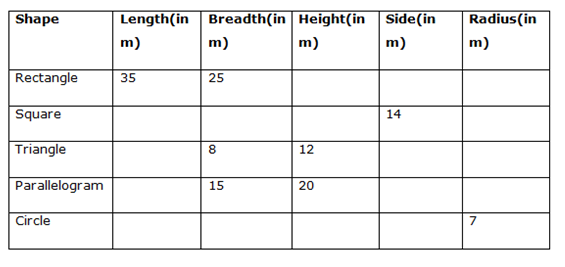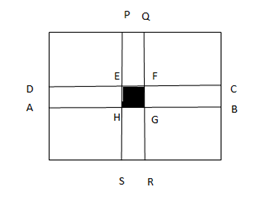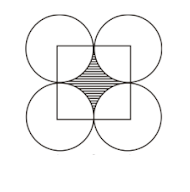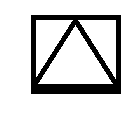# Quantitative Aptitude Questions (Data Interpretation) for SBI PO Mains 2018 Day-100

Dear Readers, SBI is conducting Online Examination for the recruitment of probationary officer. To enrich your preparation here we have providing new series of Data Interpretation – Quantitative Aptitude Questions. Candidates those who are appearing in SBI PO Mains Exams can practice these Quantitative Aptitude average questions daily and make your preparation effective.

Click “Start Quiz” to attend these Questions and view Solutions

Direction (Q.1-5): Read the following graph carefully and answer the Questions below:

 Category Total Students from school A % of boys in School  A Total boys in school in A & B together Probability of choosing 1 girl for  a committee I 800 45% 600 4/7 II 650 50% 650 68/133 III 900 45% 750 16/31 IV 550 60% 500 1/2 V 650 40% 450 16/25

1. Find the probability of selecting 2 girls to form a committee from school A and B together in category IV
1. 550/1998
2. 600/1998
3. 540/1998
4. 499/1998
5. None of these

2. Find the probability of choosing 2 boys from the entire category and which one is greater?

1. Category I
2. Category II
3. Category III
4. Category V
5. Cannot be determined
1. In how many ways a committee consisting of 3 men or 2women can be formed from category V?
1. 15456000
2. 14586000
3. 15406000
4. 16452000
5. None of these
1. If School A and School B conducts an annual function for all students. In Dance competition, 10% of total boys are participated; find the probability that boys from category III won the competition?
1. 17/59
2. 15/59
3. 44/59
4. 42/59
5. None of these
1. Find the number of ways selecting two girls from school A and B together in category II
1. 421750
2. 461720
3. 471570
4. 487580
5. None of these

Directions (Q.6-10) Study the following information carefully and answer

the given questions:1. A rectangular lawn has two roads each 10 m wide running in the middle of it, one parallel to the length and the other parallel to the breadth. Find the cost of gravelling them at Rs. 35 per square metre.
1. 22500
2. 17500
3. 25000
4. 16000
5. None of these

7. Four circles having equal radius drawn with the centres at the four corners of the square. Each circle touches other two adjacent circles. Find the cost of gravelling remaining area of the square, if it is gravelled at the rate of 25 per sq meter?

1. 1280
2. 1890
3. 1050
4. 1250
5. None of these
1. A Circular path is surrounding the circular plot is being gravelled at a total cost of Rs. 1848 at Rs. 4 per square meter. Find the width of the path.
1. 10 meter
2. 12 meter
3. 9 meter
4. 7 meter
5. None of these

9. An equilateral triangle is inscribed into the square. Find the perimeter and area of equilateral triangle.

1. 49 √3 sq m and 42 m
2. 52 √3 sq m and 36 m
3. 49 √2 sq m and 54 m
4. 52 √2 sq m and 64 m
5. None of these
1. If the breadth of the parallelogram is increased by 40 % and the height is decreased by 20 %. Find the new area of a parallelogram and also find the total cost to fence the place if the cost of fencing is Rs. 12 per meter.
1. 144 sq. m, 1295
2. 324 sq. m, 562
3. 336 sq. m, 888
4. 196 sq. m, 761
5. None of these

Direction (1-5):

 Category School A School B Total Boys Girls Total Boys Girls I 800 360 440 240 II 650 325 325 325 III 900 405 495 345 IV 550 330 220 170 V 650 260 390 190

Category I:

Probability of selecting a girl from total = (440+x)C1/(800+240+x)C1 = 4/7

(440+x)/(1040+x) =4/7

=>3080+7x=4160+4x

=>3x= 1080

=>x=360

Total students from school B in category I = 360+240= 600

Similarly find all the categories

 Category School A School B Total Boys Girls Total Boys Girls I 800 360 440 600 240 360 II 650 325 325 680 325 355 III 900 405 495 650 345 305 IV 550 330 220 450 170 280 V 650 260 390 600 190 410

(220+280)C2/ (550+450) C2

=> (500*499)/(1000*999)

=> 499/1998

= 499/1998

Category I:

(360+240)C2 / (360+440+240+360)C2 =600C2 /1400C2

= 600*599 /1400*1399 =1797 /9793

Category II:

= 650C2/1330C2 = 650*649 /1330*1329 = 42185 /176757

Category III:
=750C2 / 1550C2 = 11235/48019

Category V:

=450C2/1250C2 =4041 /31225

= (260+190) C3+ (390+410) C2= 15086400 +319600 = 15406000

Total number of boys from School A and B for all category = 2950

Number of boys participated = 2950*10/100 = 295

Number of boys in category III = 750*10/100 = 75

Probability = 75/295 = 15/59

Number of ways = 680C2

= 680*679

= 461720

Directions (Q.6-10)Length of rectangle = 35 m, Breadth = 25 m

Area is to be gravelled = Area of ABCD + Area of PQRS – Area of EFGH

= > (35*10) + (25 * 10) – (10*10)

= > 350 + 250 – 100

= > 500 Sq m

The cost of gravelling them at Rs, 35 per sq m

Total cost = 500 * 35 = Rs. 17500Here radius of the circle(r) = side of the square/2 = a/2

Remaining area of the square = a2 – πr2 = a2 – π(a/2)2

= > a2 – π(a/2)2

= > 142 – (22/7) *(7*7)

= > 196 – 154

= > 42 sq meter

The cost of gravelling = 42 * 25 =Rs. 1050

Radius of the circular plot = 7 m

Area of the circular path = 1848/4 = 462

Area of the path = π(r+x)2 – πr2 (Here x is the width of the path)

= > (22/7) [(7+x)2 – 72]

= > (22/7) [49 + 14x + x2 – 49]

= > (22/7) [14x + x2]

(22/7) [14x + x2] = 462

14x + x2 = 462 *(7/22)

14x + x2 = 147

X2 + 14x – 147 = 0

(x+21) (x – 7) =0

X = 7 meterSide of the square = 14 m = side of the triangle

Perimeter of equilateral triangle = 3a = 3*14 = 42 m

Area of equilateral triangle = (√3/4) a2

= > (√3/4) * 196

= > 49√3 sq meter

Breadth = 15, Height = 20

Area of parallelogram = bh

New area = (15*140/100)*(20*80/100)

= > 21 * 16

= > 336 sq meter

Perimeter of parallelogram = 2 (b + h)

= > 2* (21 + 16) = 74 meter

Total cost to fence the place

= > 74 * 12

= > Rs. 888

Daily Practice Test Schedule | Good Luck

 Topic Daily Publishing Time Daily News Papers & Editorials 8.00 AM Current Affairs Quiz 9.00 AM Quantitative Aptitude “20-20” 11.00 AM Vocabulary (Based on The Hindu) 12.00 PM General Awareness “20-20” 1.00 PM English Language “20-20” 2.00 PM Reasoning Puzzles & Seating 4.00 PM Daily Current Affairs Updates 5.00 PM Data Interpretation / Application Sums (Topic Wise) 6.00 PM Reasoning Ability “20-20” 7.00 PM English Language (New Pattern Questions) 8.00 PM